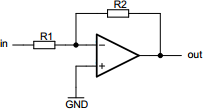# Inverting operational amplifier calculator

## Calculates properties of inverting operational amplifier circuit

 Example 1: Must calculate the output voltage of the circuit with 8 millivolt input using R1 of 2.2 kilo-ohms and R2 of 100 kilo-ohms View example
 Input voltage volts Output voltage volts Resistor R1 ohms Resistor R2 ohmsYou may use one of the following SI prefix after a value: p=pico, n=nano, u=micro, m=milli, k=kilo, M=mega, G=giga

Fill in all values except the one you wish to calculate
• Input voltage - Input voltage to op-amp
• Output voltage - Output voltage from op-amp
• Resistor R1 - Resistance of resistor R1
• Resistor R2 - Resistance of resistor R2